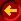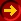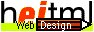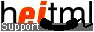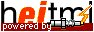# Expressions

Expressions can occur as parameters for heitml and user-defined tags. They are evaluated before execution of a specific tag.

## Data Types

heitml knows the following data types:

• Integer, 32-Bits
• Boolean, true or false
• Real, 64-Bits
• String, up to 231-1 characters
• Object
• Unknown
All types except for the object data type are described below. The object data type is described in more detail on the next page Objects.

## Values

Each value processed by heitml has one of the 6 above-described data types associated with it. Integer, Boolean, real, and string are different types. There is a difference between 6 as an integer value, "6" as a string, and 6.0 as a real value. Also, true as Boolean value and "true" as a string are different. The special value null is the only value which has the type unknown.

## Variables

Each heitml variable has an identifier, which must be declared when a value is first assigned to it. heitml is a Dynamically Typed Language, which means that variable types are assigned according to the type of data associated with it. Later, the variable type can change simply by assigning it a new type of data.

Variables are local to the current heitml-page or to the current invocation of a procedure.

heitml also provides the concept of Global Variables which means that all procedures can access these variables.

## Assignments

Assignments occur when explicitly written down with the = operator, when using the assign, dbquery, for or forin tag, and implicitly during parameter passing.

Assignments of all types except object have the usual value semantics, the whole value is copied. For objects, pointer semantics applies. This means an object is allocated on the heap and an object variable always contains a reference. Assignment assigns this reference.

## Operations

heitml knows the operators + (add), - (subtract), * (multiply), / (divide), % (modulo) which work as usual on integer and real operands. The plus symbol + can also be used to concatenate strings. Using the minus symbol - as an unary operator, numeric values are negated.
 Syntax: Expr::= Expr + Expr | Expr - Expr | Expr * Expr | Expr / Expr | Expr % Expr | - Expr .

Binary Boolean expressions use the operators ==, !=, <, <=, &lt, &gt, &le, &ge, ||, &&. Note that there is no > operator for syntactical reasons. &lt stands for less than, &le for less or equal, &gt for greater than, and &ge for greater or equal.
 Syntax: Expr::= Expr == Expr | Expr != Expr | Expr && Expr | Expr || Expr | Expr < Expr | Expr < Expr | Expr < Expr | Expr <= Expr | Expr &le Expr | Expr ≤ Expr | Expr > Expr | Expr > Expr | Expr &ge Expr | Expr ≥ Expr .

Unary Boolean expressions consist of the not operator ! followed by a Boolean expression.
 Syntax: Expr::= ! Expr .

The assignment operator = assigns the right operand to the left operand and returns the result of the right operand.
 Syntax: Expr::= Expr = Expr .

Therefore, the left operand must denote a variable or a field of an object.

The operator , evaluates both operands and returns the result of the second operand.
 Syntax: Expr::= Expr , Expr .

Note that expression with the operators = and , must be parenthesized within the expression of the ? tag, the condition of the while and if statement, within the operator [ ], in parameters of a function call and default values of function parameters.
 Syntax: Expr::= ( Expr ) .

The operator [ ] is used to index an object or a string. In the case of an object, the operator [ ] is used to access an object field or to call a method of an object.
 Syntax: Expr::= Expr [ Expr ] | Expr [ Expr ] ( ActualParameterList ) .

If a string s is indexed through s[i], the index i must be of type integer denoting the i-th character of s, where counting starts with 0. For indexing an object with the [ ] operator, refer to the Objects page.

The operator . is used to access an object field or to call a method of an object.
 Syntax: Expr::= Expr . Fieldname | Expr . MethodName ( ActualParameterList ) . FieldName::= Identifier .

Finally, an operation maybe a function call.
 Syntax: Expr::= FunctionName ( ActualParameterList ) .

### Operator Precedence

Operator operands may contain expressions called sub-expressions, so expressions have a tree structure with the root at the top. When an expression evaluated, there is a certain order to how it is done. Operands are always evaluated first, so the tree is evaluated from the leaves to the root. Unfortunately, the tree structure of an expression is not unique on user input. For example, the expression 2*2+2 may be evaluated to 8 if 2 and 2+2 are considered as the operands of the * operator or it may be evaluated to 6 if 2*2 and 2 are considered as the operands of the + operator. To make expressions unique, operators have precedence over other operators. The operator precedence is defined below. In our example, the * operator has precedence over the + operator and so the expression is evaluated to 6. The user can overrule the default operator precedence, by parenthesizing sub-expressions. For example, to evaluate the previous expression to 8, the user would have to write the expression as 2*(2+2).

The following list shows the operators with ascending precedence:
, = || && == != < <= &ge &gt &le &lt + - * / % unary - ! . [ ] ( ).

### Type checking

The type of an expression type is determined by its constituents. If an expression is a constant or variable, the type of the expression is the type of the constant or variable. If an expression consists of an operator with its operands the type of the expression is the type of the operators result which itself may depend on the type of the operands. For example, expressions consisting of comparison operators (also called conditions) are independent of the type of the operands and always will result in value of type Boolean. In contrast, expressions with a + operator have a type dependent on the type of the operands. The type of the expression 2+2 is integer and the type of "a"+"b" is a string.

Except for the operators =, ,, ==, and !=, the type of the left operand and the right operand must be the same. If necessary, the following coercions are done on the operands:

Coercion onleft operandCoercion onright operand
Real (Integer )Real
RealReal (Integer )
StringString (Integer )
String (Integer )String
StringString (Real )
String (Real )String

The following table summarizes which operators expect which operand types. A '*' entry means that the operator does not care about operand types. All other combinations result in the error message "Type error in expression".

Operatortype of left operandtype of right operandResult type
, =*type of right operand
== !=*Boolean
|| &&BooleanBoolean
< <= &ge &gt &le &ltReal, Integer, String, BooleanBoolean
+Real, Integer, StringReal, Integer, String, respectively
- * / %Real, IntegerReal, Integer, respectively
unary -Real, Integernot applicableReal, Integer, respectively
!Booleannot applicableBoolean
.Object*type of object field or result of method call
[ ]ObjectString, Integertype of object field or result of method call
StringIntegerString
( )*not applicable*

This page was dynamically generated by the web application development tool RADpage of H.E.I. H.E.I. provides support, tools, and services like Webdesign in Mannheimm, the HTML/CSS 3D WebGL Animation Library taccgl, 3D Webdesign, and 3D Product Configurator (3D Produkt Konfigurator in German).

Selected blog articles : 3D Objects on HTML pages, CSS Transition Visibility, and CSS Transition Display.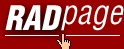Homepage Intro/Features Component Guide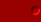Programming Language Guide Language Ref. General Design Lexical Structure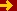Expressions Objects Methods Classes heitml Tags heitml Functions Advanced Functions Database Access Global Variables Form Fields Server Variables Sessions heitml Syntax Component Ref. Class Library User Components Tutorial New Features heitml 1User Guide Services Privacy DatenschutzContact / Impressum